11 篇文章 0 订阅

# 深入理解SVM

martin

## SVM核心思想一：最大间隔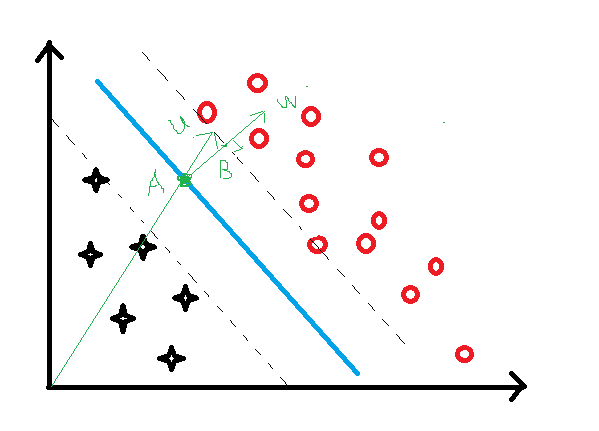wx++b>=1

wx+b<1

## SVM核心思想二：决策公式

y $y$的取值给出：

y={+1x-1x

wx++b>=1

wx+b<1

yi(wxi+b)1>=0

yi(wxi+b)1=0

## SVM核心思想三：目标函数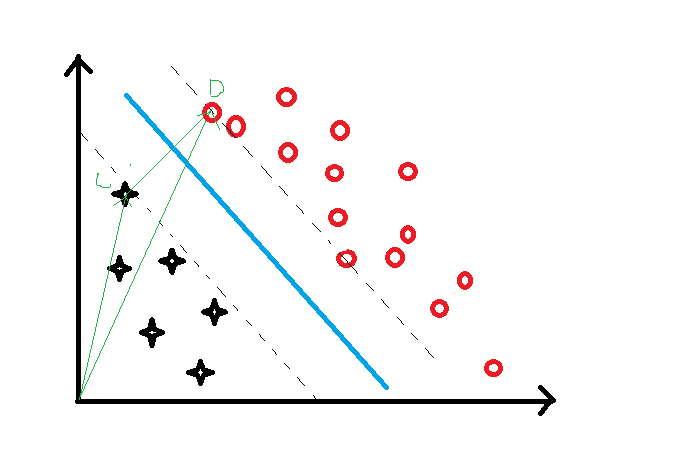=ODOC=(x+x)ww

yi(wxi+b)1=0

=1w(wx+wx)=1w[(1b)+(b+1)]=2w

max 2w

max 1w

min w

min 12w2

## SVM核心思想四：优化理论

L=12w2i=1nαi[yi(wxi+b)1]

Lw=wi=1nαiyixi

wi=1nαiyixi=0

w=i=1nαiyixi

Lb=i=1nαiyi

i=1nαiyi=0

i=1nαiyi=0

L=i=1nαi12i=1nj=1nαiαjyiyjxixj

min L=i=1nαi12i=1nj=1nαiαjyiyjxixj

wxtest+b=0

f=sign(wxtest+b)

## SVM核心思想五：损失函数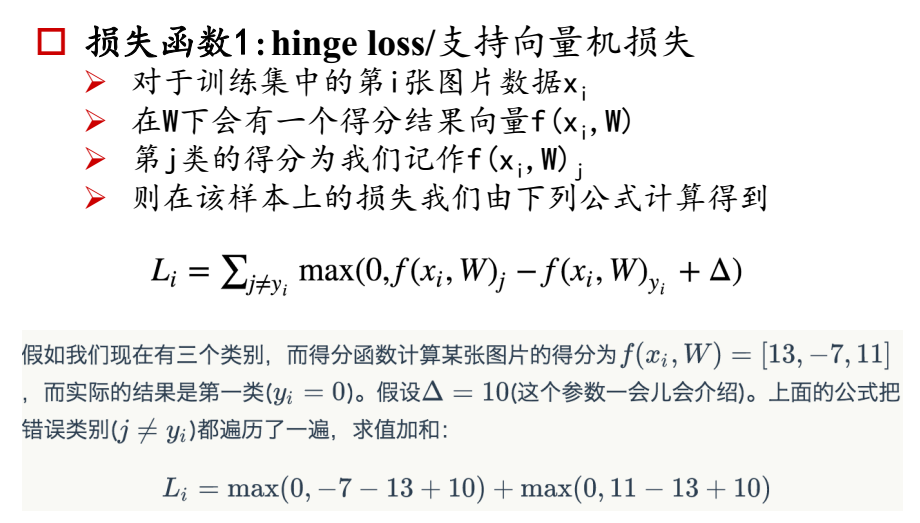Li=jyimax(0,f(xi,W)jf(xi,W)yi+Δ)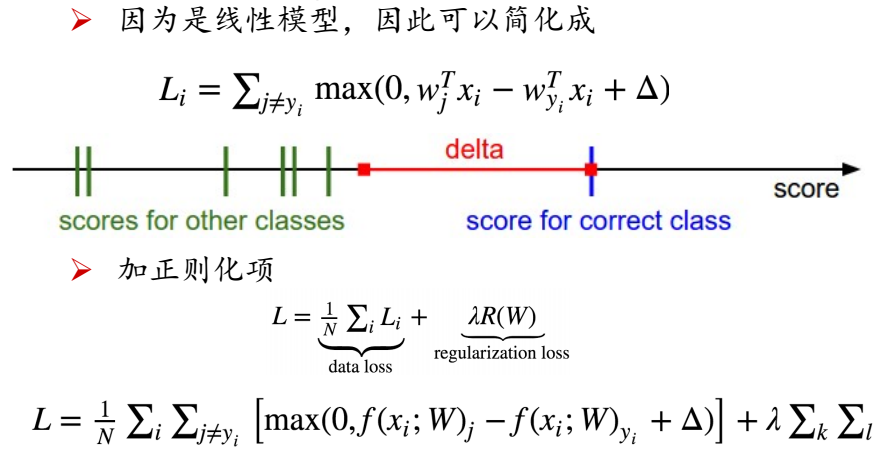## SVM核心思想六：核方法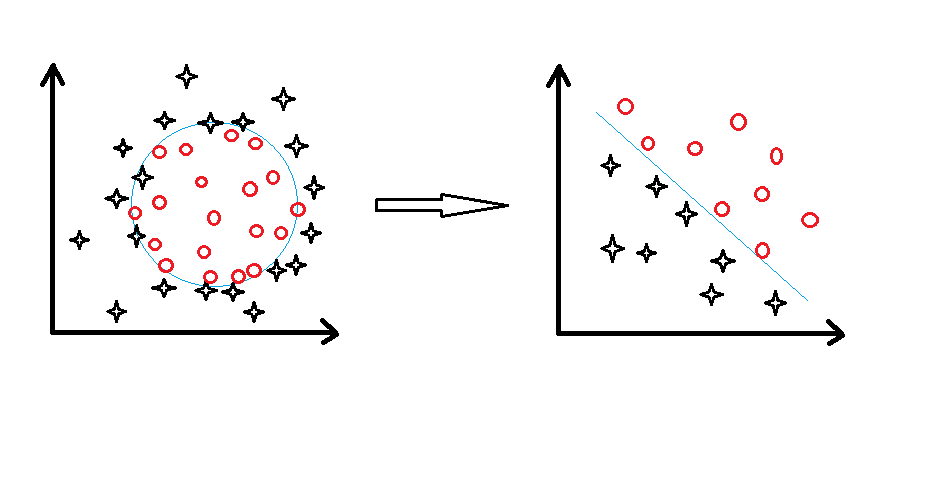## SVM核心思想七：SMO

min L=i=1nαi12i=1nj=1nαiαjyiyjxixj

• 1、如果所有变量都满足最优化问题的KTT条件那么就会得到最优解，
• 2、否则选择两个变量，固定其他变量，针对这两个变量构建二次规划问题。

$\color{red}{注意：}$子问题的两个变量中只有一个是自由变量。假设 α1,α2 $\alpha_1,\alpha_2$为两个变量， α3,α4,...αN $\alpha_3,\alpha_4,...\alpha_N$固定，那么：

α1=y1i=2Nαiyi

02-112万+
04-187万+04-16256
06-078175
07-278万+
06-05200
10-081万+
03-061万+
10-23116
04-12
02-252885
01-201709
04-05188
12-131万+
04-221858
01-0158
05-29
04-271万+
03-038291点击重新获取扫码支付余额充值